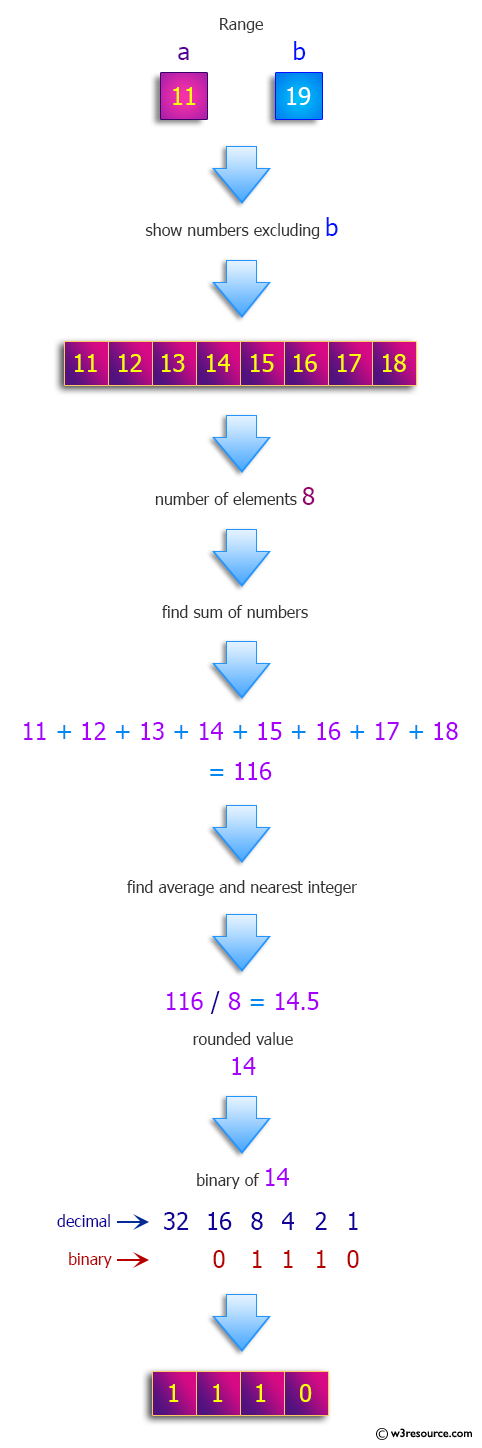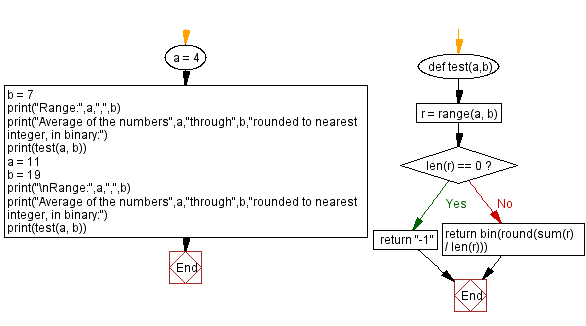﻿ Python: Calculate the average of the numbers a through b rounded to nearest integer, in binary - w3resource# Python: Calculate the average of the numbers a through b rounded to nearest integer, in binary

## Python Programming Puzzles: Exercise-81 with Solution

Write a Python program to calculate the average of the numbers a through b ( b not included ) rounded to nearest integer, in binary (or -1 if there are no such numbers).

```Input:
4 , 7
Output:
0b101

Input:
11 , 19
Output:
0b1110
```

Pictorial Presentation:Sample Solution:

Python Code:

``````#License: https://bit.ly/3oLErEI

def test(a,b):
r = range(a, b)
if len(r) == 0:
return "-1"
return bin(round(sum(r) / len(r)))

a = 4
b = 7
print("Range:",a,",",b)
print("Average of the numbers",a,"through",b,"rounded to nearest integer, in binary:")
print(test(a, b))
a = 11
b = 19
print("\nRange:",a,",",b)
print("Average of the numbers",a,"through",b,"rounded to nearest integer, in binary:")
print(test(a, b))
``````

Sample Output:

```Range: 4 , 7
Average of the numbers 4 through 7 rounded to nearest integer, in binary:
0b101

Range: 11 , 19
Average of the numbers 11 through 19 rounded to nearest integer, in binary:
0b1110
```

Flowchart:## Visualize Python code execution:

The following tool visualize what the computer is doing step-by-step as it executes the said program:

Python Code Editor :

Have another way to solve this solution? Contribute your code (and comments) through Disqus.

What is the difficulty level of this exercise?

Test your Programming skills with w3resource's quiz.

﻿

## Python: Tips of the Day

Clamps num within the inclusive range specified by the boundary values x and y:

Example:

```def tips_clamp_num(num,x,y):
return max(min(num, max(x, y)), min(x, y))
print(tips_clamp_num(2, 4, 6))
print(tips_clamp_num(1, -1, -6))
```

Output:

```4
-1
```